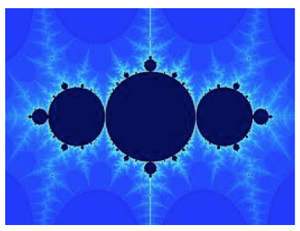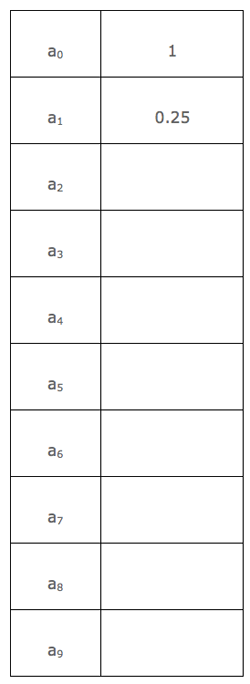Te Kete Ipurangi
Communities
Schools

### Te Kete Ipurangi user options:

Activities:

Activities:

Maths activities:

Stats activities:

# Activity: The Big Bang Theory - Fractals and complex numbers

## Purpose

To explore complex numbers in the context of the Julia and Mandelbrot Sets.

## Achievement objectives

• M 8-9 Manipulate complex numbers and present them graphically
• M 8-10 Identify discontinuities and limits of functions
• M 8-2 Display and interpret the graphs of functions with the graphs of their inverse and/or reciprocal functions

## Indicators

• Uses and manipulates surds and other irrational numbers.
• Interprets and uses graphical representations of complex numbers, using polar and rectangular form on an Argand diagram.
• Uses and manipulates complex numbers, making links with their graphical representation.
• Links features of graphs with the limiting behaviour of functions.
• Uses limiting features of functions to sketch graphs.
• Demonstrates understanding of inverse and/or reciprocal functions, including those of:
• polynomial
• Finds limits algebraically, graphically, and numerically by considering behaviour as:
• x approaches a specific value from above and below
• x tends towards +∞ or -∞

## Specific learning outcomes

Students will be able to:

• Solve problems that require an extended chain of reasoning.
• Understand and iterate functions.
• Manipulate real and complex numbers.
• Use Argand diagrams.
• Simplify sums, differences, products and quotients of surds.
• Simplify sums, differences, products and quotients of complex numbers expressed in rectangular form.
• Sketch the graph of a parabola
• Solve problems involving points of intersection between lines and conics.

## Diagnostic snapshot(s)Diagnostic snapshot

A fractal is a fragmented geometric shape that can be split into parts, each of which is a reduced-size copy of the whole. Roots of the idea of fractals go back to the 17th Century, however, the term fractal was coined by Benoît Mandelbrot in 1975. A mathematical fractal is based on an equation that undergoes iteration.

Guiding questions:

• Can you draw the function f(x) = x2 – 3.25?
• Can you find f(1), f(5), f(-3) and f(3 + 2i)?
• What does i represent?

## Planned learning experiences

### Starter

Watch the first seven minutes of The Big Bang Theory, Season 1, Episode 2.

(This link is to a YouTube clip. As the link may not be maintained, it may be more appropriate to buy or rent the DVD to show the class.)

Use Google images to find some more Mandelbrot and Julia Fractals.

And now for the maths:

• Take: f(x) = x2 – 0.75
• Find f(1). This is a1.
• Find f(a1). This is a2.
• Find f(a2). This is a3.Chart

• Describe this sequence of numbers.
• Is the sequence approaching any particular value?
• What has this got to do with fractals?

### Learning activity

Starting a fractal:

1. Draw the curve y = x2 – 0.75 on a set of axes between -2 and 2 in red.
2. Draw the line y = x in blue.
3. Mark your a  to a9 values along the x-axis.
4. Join your a  value to the red curve with a vertical line, from this point go horizontally across until you meet the blue line. Come down from this point to the x-axis. This should be your a1 value.
5. Join the a1 value to the red curve with a vertical line, and continue the pattern. This begins your fractal. The more iterations you do, the more ‘fractal like’ it becomes.

#### Experiment 1

• With f(x) = x2 – 0.75 try different starting values of a . For example, try 0.5, 0.8, 1.2, 1.8 and 2.

#### Experiment 2

• Try a different base function. It can be from the same family of graphs, or a different one. What do you notice?

#### Experiment 3

• Try a sample function within a complex number framework: f(z) = z2 – 0.75, your a  value is i.
• What happens here? How many iterations do you have to do to decide the outcome?
• Try other imaginary numbers (for example, 0.5i) as your a0. Plot this on an Argand diagram.
• Try some complex numbers (for example, 1/2 + 3i/4) as your a .

• Physics

## Extension/enrichment ideas

• Investigate multiple base functions.
• Investigate fractal types.
• Investigate the history of Benoit Mandelbrot or another modern Mathematician.

## Planned assessment

This teaching and learning activity could lead towards assessment in the following achievement standard:

## Spotlight on

### Pedagogy

• Creating a supportive learning environment:
• Accepting all student responses as part of the learning process.
• Expecting all students to be able to do mathematics and statistics with understanding.

### Key competencies

• Thinking:
• Students select appropriate methods and strategies when solving problems.
• Students make deductions, they justify and verify, interpret and synthesise and they create models.
• Students hypothesise, investigate, analyse and evaluate.
• Using language, symbols and texts:
• Students use symbols and diagrams to solve problems.
• Relating to others:
• Students listen to others, they accept and value different viewpoints.
• Students work in groups, they debate solutions, negotiate meaning and communicate thinking.
• Managing self:
• Students develop skills of independent learning.
• Students are prepared to take risks, make decisions, and persevere.
• Participating and contributing:
• Students contribute to a culture of inquiry and learning. They share strategies and thinking and they empower and enable others.

### Values

Students will be encouraged to value:

• Innovation, inquiry and curiosity by thinking critically, creatively, and reflectively
• Community and participation for the common good

### Māori/Pasifika

• Ka Hikitia
• Pasifika Education Plan
• It may be appropriate to investigate whether there are other Mathematicians in different cultures who have investigated fractals since the 17th century.

### Planning for content and language learning

• ESOL Online
• Reinforce vocabulary such as function, iteration etc.